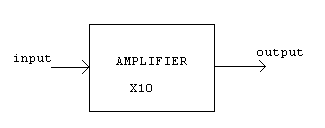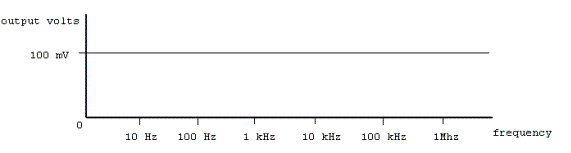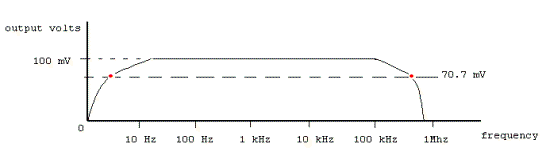Electronic Circuits and Tutorials
Tutorials
Dictionaries
Projects
Discover
Home > Electronics Tutorial > Test and Measurement > Frequency Response Tutorial
Test and Measurement in Electronics

# Frequency Response TutorialA perfect amplifier with an amplification of times 10, as shown above, would give an output 10 times greater than the input, NO MATTER WHAT THE INPUT FREQUENCY.

If the input was 10 mV then the output would be 100 mV, no matter the frequency of the input signal, as seen in the graph below. This graph is known as a FREQUENCY RESPONSE diagram.In a practical amplifier it is not possible to obtain a perfectly flat response curve. This is due to limitations of electronic components and circuitry. Usually there is a fall of response at low and high frequencies.The two points in red on the response curve mark where the output of the amplifier has fallen to 70.7 % of the maximum output. This means that that the 100mV output has fallen to 70.7 mV at these frequencies. These are called the -3 dB points.

One is at about 5Hz (call it f1). The other is at about 900 kHz (f2).

Subtract f1 from f2 to get the BANDWIDTH of the amplifier.

In this case it is just under 900 kHz wide.

In some cases the bandwidth is tailored to pass some frequencies and not others.

This is called FILTERING

Note: To report broken links or to submit your projects, tutorials please email to Webmaster